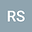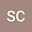On Stability and hyperstability of Drygas functional equation in quasi-Banach space
••• Ravinder Sharma,
• Sumit Chandok
Ravinder Sharma
Thapar Institute of Engineering and Technology
Author ProfileSumit Chandok
Thapar Institute of Engineering and Technology
Author Profile## Abstract

In this paper, we investigate the Ulam-Hyers-Rassias stability for the Drygas functional equation $f( x + y ) + f( x - y ) = 2f (x) + f (y)+ f(-y)$ in the setting quasi-Banach spaces using the approach of Brzdek’s fixed point theorem. Also, we gave a general result on the hyperstability of Drygas functional equation. The results obtained in this paper extend various previously known results to the setting of quasi-Banach space.